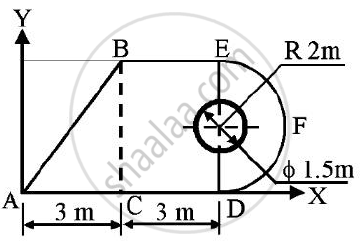Advertisement Remove all ads

# A Circle of Diameter 1.5 M is Cut from a Composite Plate.Determine the Centroid of the Remaining Area of Plate. - Engineering Mechanics

Answer in Brief

A circle of diameter 1.5 m is cut from a composite plate.Determine the centroid of the remaining area of plate.Advertisement Remove all ads

#### Solution

 PART AREA( in m2) X co-ordinate of centroid(m) Y co-ordinate of centroid(m) Ax(m3) Ay(m3) Rectangle 3 x 4=12 3 + 3/2 = 4.5 2 54 24 Triangle 0.5 x 3 x 4=6 3 - 3/3 = 2 4/3 = 1.3333 12 8 Semicircle 0.5 x 22 x 𝝅=6.2832 6+(4 xx 2)/(3π)=6.8488 2 43.0324 12.5664 Circle(To be removed) -𝝅r2 =-0.752 x 𝝅 =-1.7671 6 2 -10.6029 -3.5343 Total 22.5161 98.4295 41.0321

X co-ordinate of centroid ( 𝑥̅ ) = (ΣAx)/(ΣA) = (98.4295)/(22.5161) = 4.3715 m
Y co-ordinate of centroid(𝑦̅) = (ΣAy)/(ΣA) = (41.0321)/(22.5161) = 1.8223 m

Centroid is at (4.3715,1.8223)m

Concept: Velocity and Acceleration in Terms of Rectangular Co-ordinate System
Is there an error in this question or solution?
Advertisement Remove all ads

#### APPEARS IN

Advertisement Remove all ads
Advertisement Remove all ads
Share
Notifications

View all notifications

Forgot password?